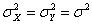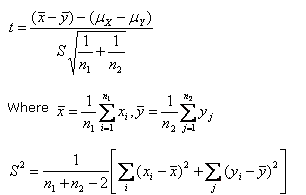# Statistics Assignment Help With T Test For Difference Of MeansSuppose we want to test if two independent samples xi (i=1, 2, …….n1) and yj (j =1,2…………n2) of sizes n1 and n2 have been drawn from two normal populations with means μX and μY respectively.

Under the null hypothesis (H0) that the samples have been drawn from the normal populations with means μX and μY and under the assumption that the population variance are equal that is, the statistic

###Is an unbiased estimate of the common population variance σ2, follows Student’s t – distribution with (n1 + n2 – 2) degrees of freedom.